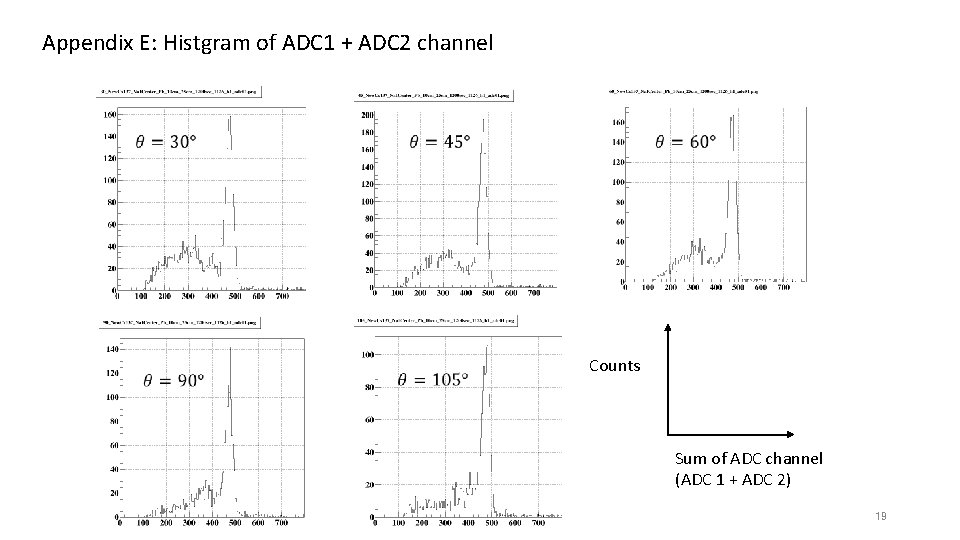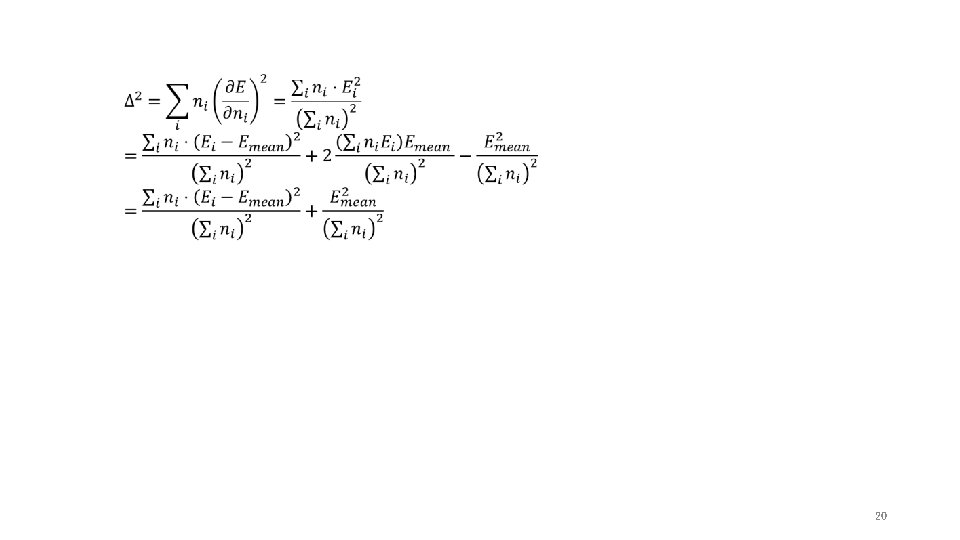# Compton scattering and KleinNishina formula Shibata lab 1214594

• Slides: 21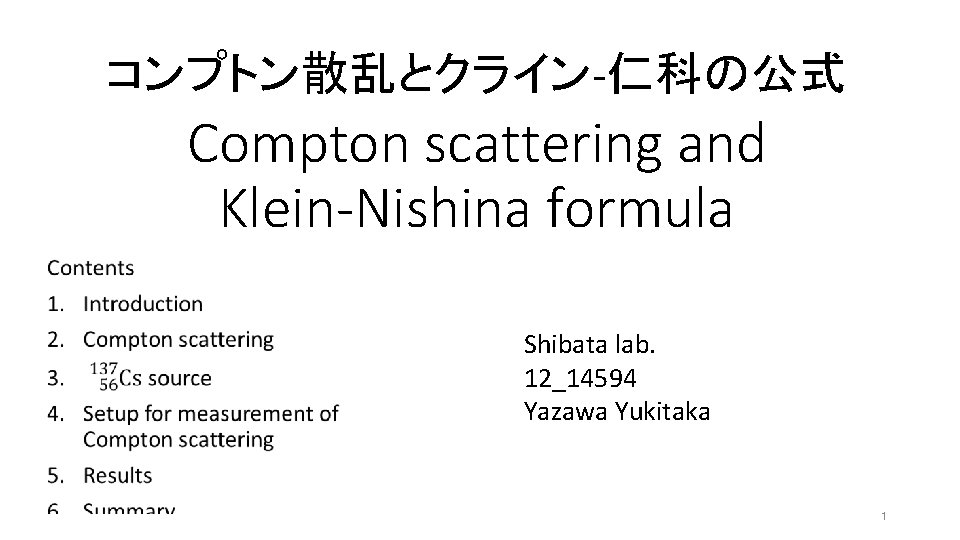コンプトン散乱とクライン-仁科の公式 Compton scattering and Klein-Nishina formula Shibata lab. 12_14594 Yazawa Yukitaka 1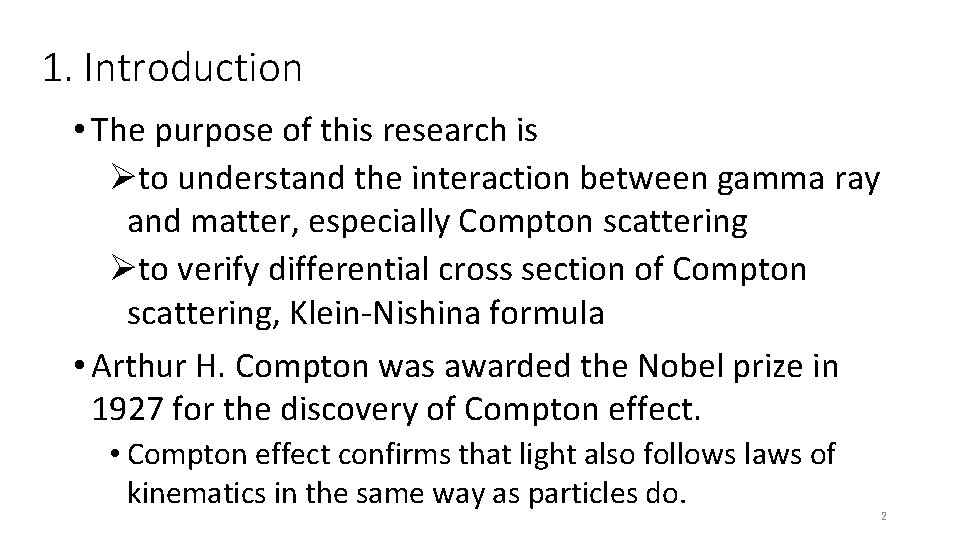1. Introduction • The purpose of this research is Øto understand the interaction between gamma ray and matter, especially Compton scattering Øto verify differential cross section of Compton scattering, Klein-Nishina formula • Arthur H. Compton was awarded the Nobel prize in 1927 for the discovery of Compton effect. • Compton effect confirms that light also follows laws of kinematics in the same way as particles do. 22. Compton scattering Law of energy and momentum conservation : Momentum : Energy : Lorentz factor Energy [ke. V] Energy of incident photon: 662 ke. V Energy of scattered photon Energy of recoil electron 662 ke. V 3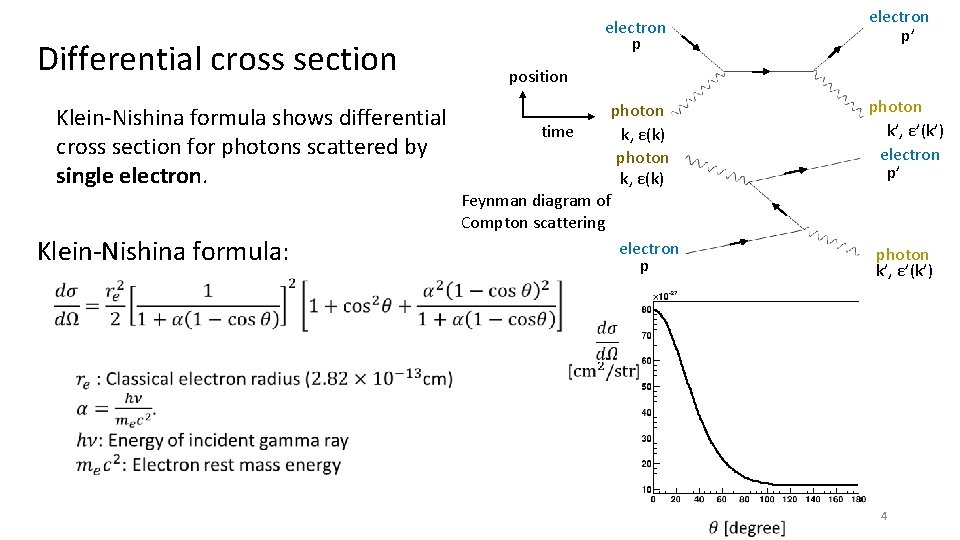Differential cross section Klein-Nishina formula shows differential cross section for photons scattered by single electron p’ electron p position time photon k’, ε’(k’) electron p’ photon k, ε(k) Feynman diagram of Compton scattering Klein-Nishina formula: electron p photon k’, ε’(k’) 4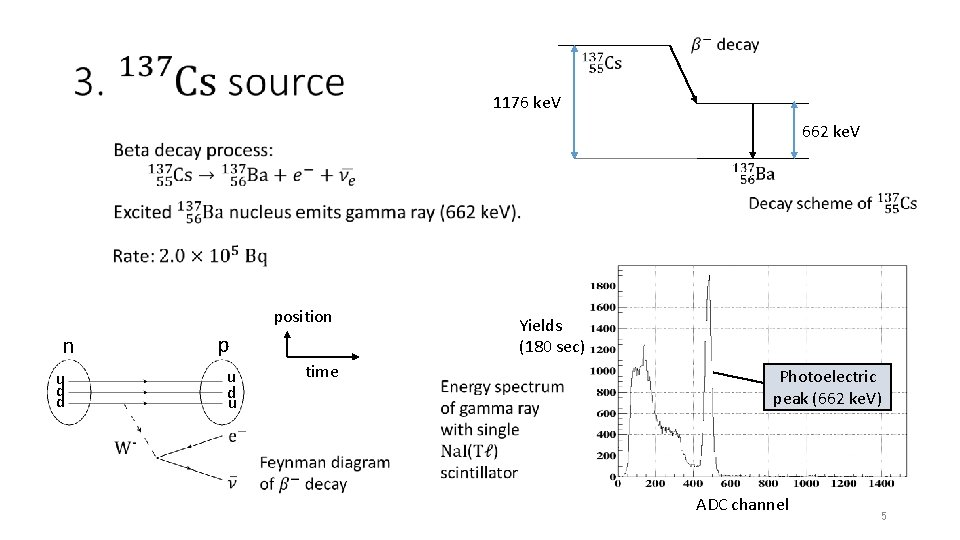1176 ke. V 662 ke. V position Yields (180 sec) p n time u d u u d d Photoelectric peak (662 ke. V) ADC channel 5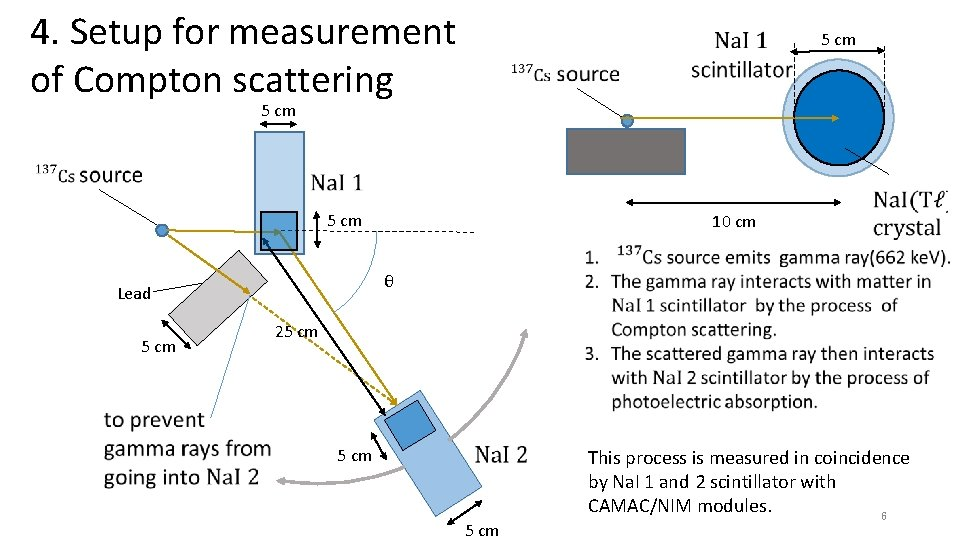4. Setup for measurement of Compton scattering 5 cm 10 cm θ Lead 5 cm 25 cm This process is measured in coincidence by Na. I 1 and 2 scintillator with CAMAC/NIM modules. 6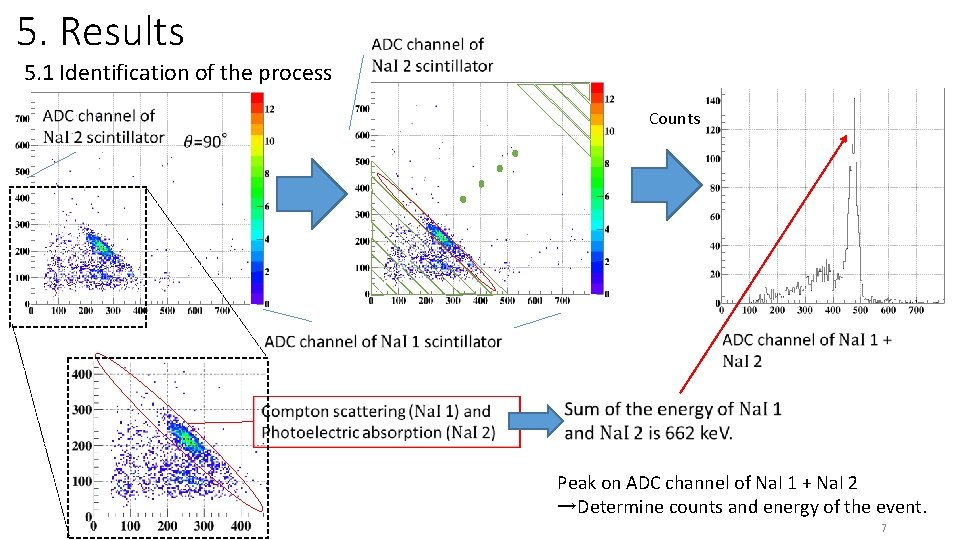5. Results 5. 1 Identification of the process Counts cc cc cc Peak on ADC channel of Na. I 1 + Na. I 2 →Determine counts and energy of the event. 7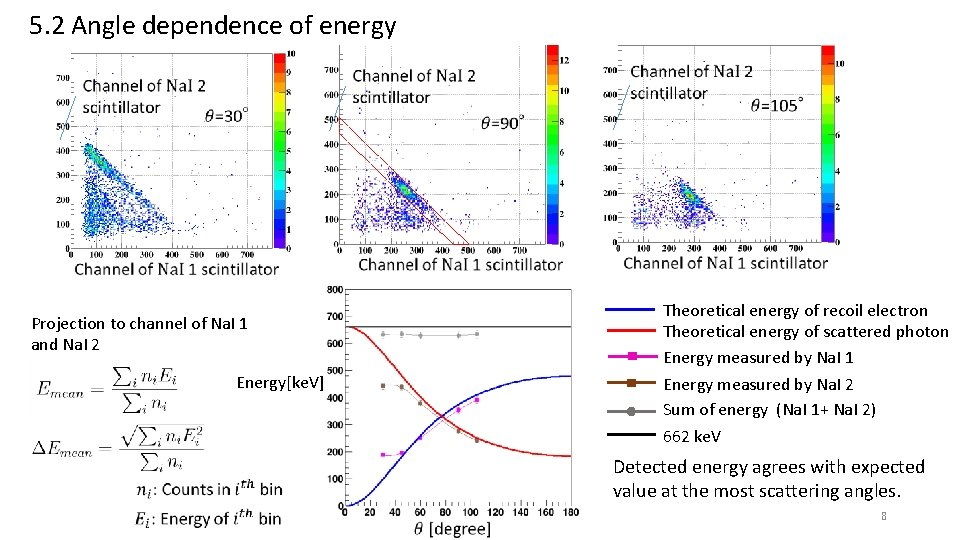5. 2 Angle dependence of energy Theoretical energy of recoil electron Theoretical energy of scattered photon Energy measured by Na. I 1 Projection to channel of Na. I 1 and Na. I 2 Energy[ke. V] Energy measured by Na. I 2 Sum of energy (Na. I 1+ Na. I 2) 662 ke. V Detected energy agrees with expected value at the most scattering angles. 8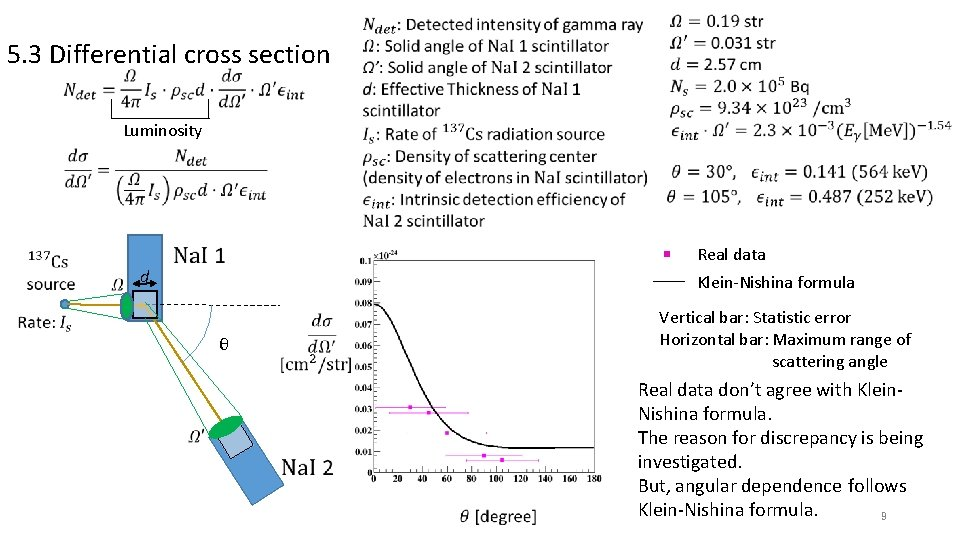5. 3 Differential cross section Luminosity Real data d Klein-Nishina formula θ Vertical bar: Statistic error Horizontal bar: Maximum range of scattering angle Real data don’t agree with Klein. Nishina formula. The reason for discrepancy is being investigated. But, angular dependence follows Klein-Nishina formula. 9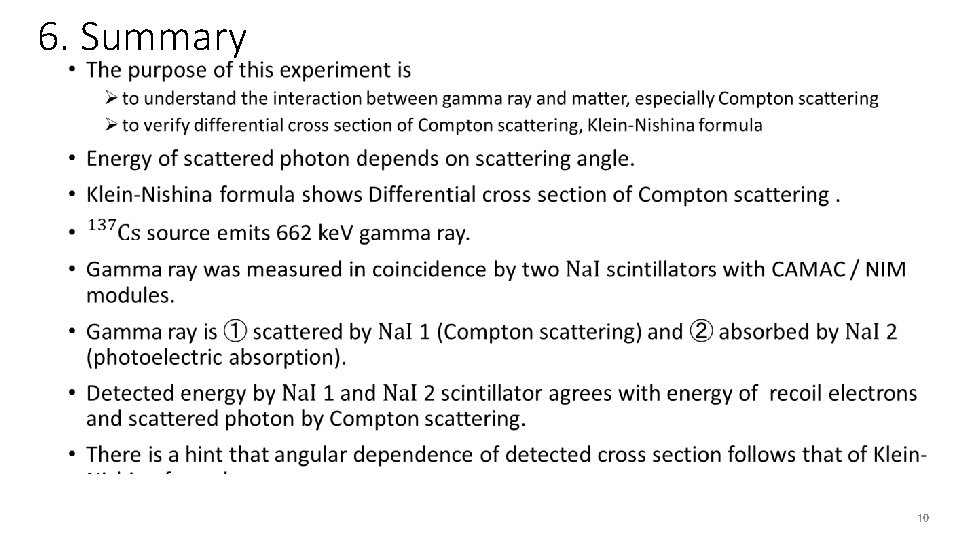6. Summary • 1012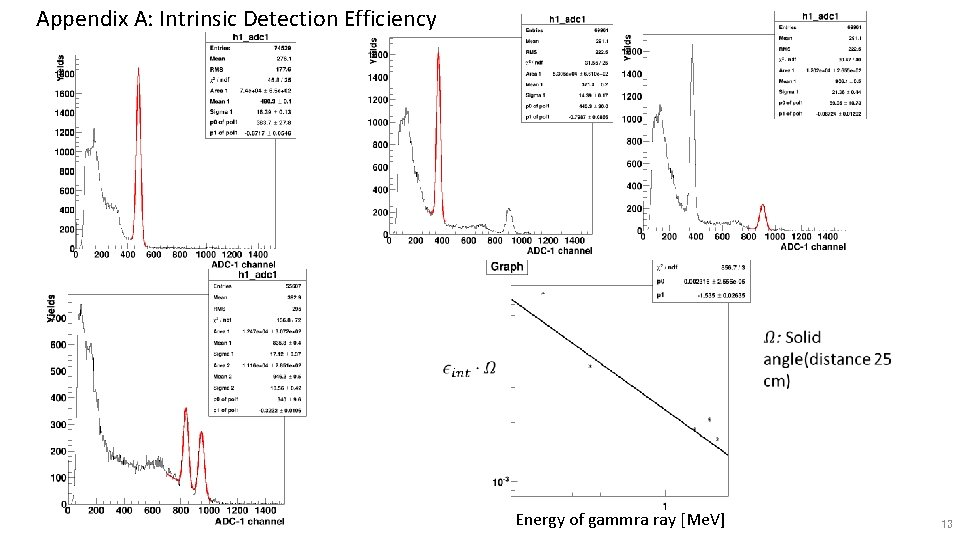Appendix A: Intrinsic Detection Efficiency Energy of gammra ray [Me. V] 13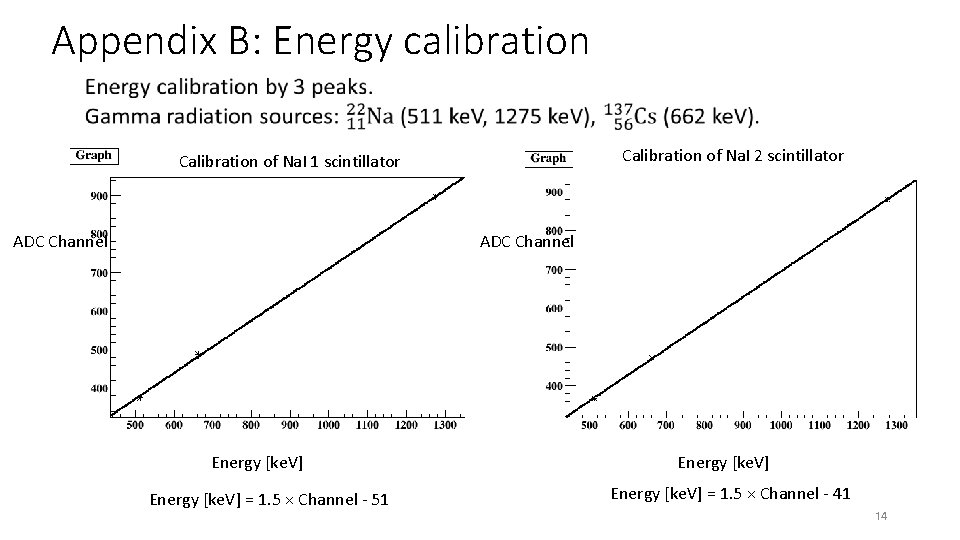Appendix B: Energy calibration Calibration of Na. I 2 scintillator Calibration of Na. I 1 scintillator ADC Channel Energy [ke. V] = 1. 5 × Channel - 51 Energy [ke. V] = 1. 5 × Channel - 41 14Appedix B: Energy Calibration 2 Na. I scintillator 2 Na. I scintillator 1 ADC channel Energy of gamma ray [ke. V] Energy [ke. V] = 1. 3 × Channel – 4. 4 Energy of gamma ray [ke. V] Energy [ke. V] = 1. 3 × Channel + 38 15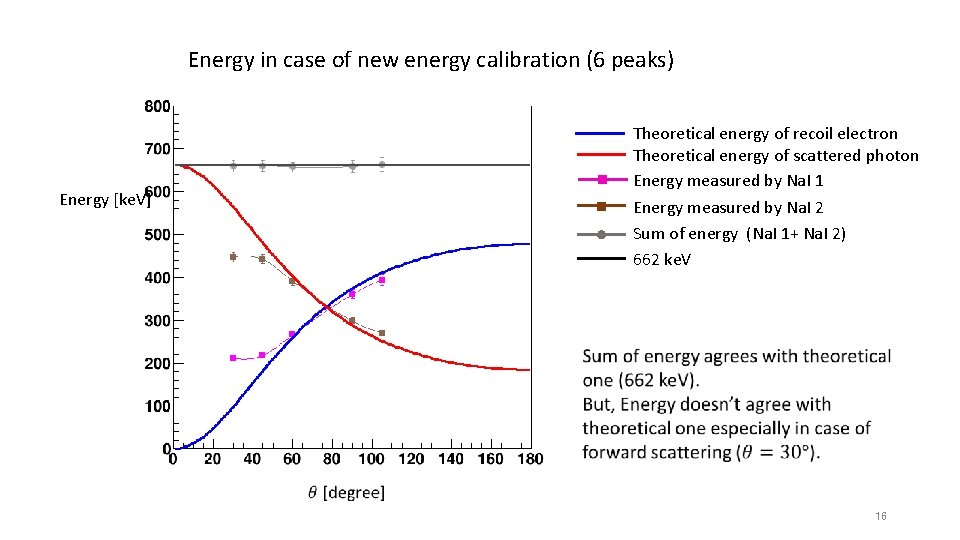Energy in case of new energy calibration (6 peaks) Theoretical energy of recoil electron Theoretical energy of scattered photon Energy measured by Na. I 1 Energy [ke. V] Energy measured by Na. I 2 Sum of energy (Na. I 1+ Na. I 2) 662 ke. V 16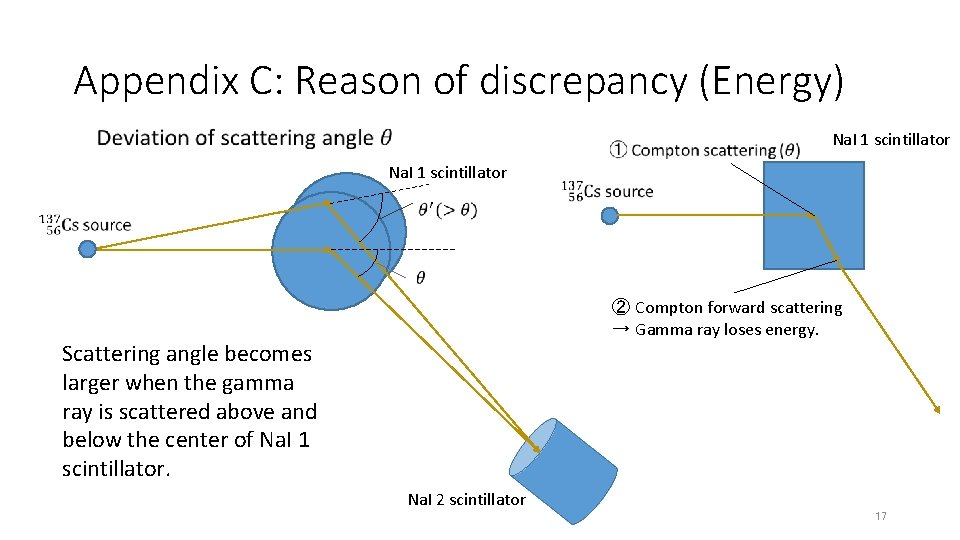Appendix C: Reason of discrepancy (Energy) Na. I 1 scintillator ② Compton forward scattering → Gamma ray loses energy. Scattering angle becomes larger when the gamma ray is scattered above and below the center of Na. I 1 scintillator. Na. I 2 scintillator 17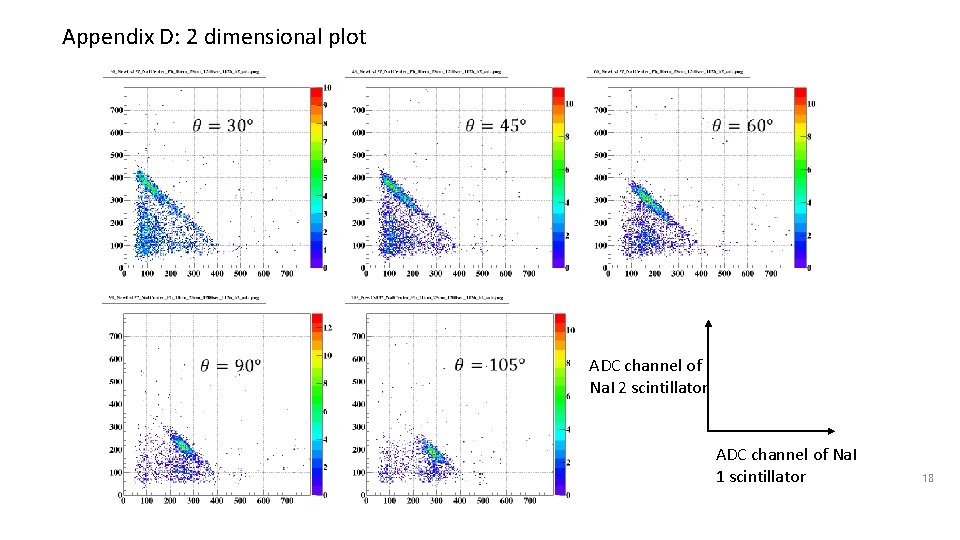Appendix D: 2 dimensional plot ADC channel of Na. I 2 scintillator ADC channel of Na. I 1 scintillator 18